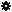﻿ Object Model: NurbsCurve.EvaluatePosition

NurbsCurve.EvaluatePosition

## NurbsCurve.EvaluatePosition### Description

Returns a 3D vector containing the position, tangent in U direction, normal and bi-normal at a given U value.

### C# Syntax

 ```Object NurbsCurve.EvaluatePosition( Double in_dUValue ); ```

### Scripting Syntax

 ```oArray = NurbsCurve.EvaluatePosition( UValue ); ```

### Return Value

A 1-dimensional Array containing the position, tangent, normal and bi-normal.

### Parameters

Parameter Type Description
UValue Double The U Value at which we want to evaluate the curve.

### Examples

#### 1. VBScript Example

 ```set oRoot = Application.ActiveProject.ActiveScene.Root set oArc = oRoot.AddGeometry( "Arc", "NurbsCurve" ) aValues = oArc.ActivePrimitive.Geometry.Curves(0).EvaluatePosition( 3.0 ) set oPosition = aValues(0) logmessage "The position at 3.0 is x :" & oPosition.x & " y: " _ & oPosition.y & " z: " & oPosition.z set oUTangent = aValues(1) logmessage "The tangent in U at 3.0 is x :" & oUTangent.x & " y: " _ & oUTangent.y & " z: " & oUTangent.z set oNormal = aValues(2) logmessage "The normal at 3.0 is x :" & oNormal.x & " y: " & oNormal.y _ & " z: " & oNormal.z set oBiNormal = aValues(3) logmessage "The bi-normal at 3.0 is x :" & oBiNormal.x & " y: " _ & oBiNormal.y & " z: " & oBiNormal.z ' Expected result: 'INFO : The position at 3.0 is x :1.16113870901785 y: 3.82776134292884 z: 0 'INFO : The tangent in U at 3.0 is x :0.956940009306386 y: -0.290285753334009 z: 0 'INFO : The normal at 3.0 is x :-0.290285753334009 y: -0.956940009306387 z: 0 'INFO : The bi-normal at 3.0 is x :0 y: 0 z: -1 ```

#### 2. JScript Example

 ```/* See also the JScript example for NurbsCurveList.GetClosestCurvePosition2 which demonstrates how to use NurbsCurveList.GetClosestCurvePosition2 and NurbsCurve.EvaluatePosition as an (object model) alternative to the EvaluateCurveAt command. */ ```